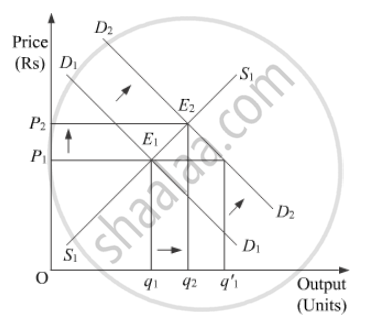Share

# Market for a Good is in Equilibrium. There is an ‘Increase’ in Demand for this Good. Explain the Chain of Effects of this Change. Use Diagram. - Economics

#### Question

Market for a good is in equilibrium. There is an ‘increase’ in demand for this good. Explain the chain of effects of this change. Use diagram.

#### Solution

Market equilibrium is a state or a position where market demand equals market supply. Now, if the market demand increases, then it results in a change in the equilibrium.Suppose D1D1 and S1S1 are the initial market demand curve and the initial market supply curve, respectively. The initial equilibrium is established at point E1, where the market demand curve and the market supply curve intersects each other. Accordingly, the equilibrium price is OP1 and the equilibrium quantity demanded is Oq1.

Now, if there is an increase in the market demand, the market demand curve shifts parallely rightwards to D2D2 from D1D1, while the market supply curve remains unchanged at S1S1. This implies that at the initial price OP1, there exist excess demand equivalent to (Oq'1 − Oq1) units. This excess demand will increase competition among the buyers and they will now be ready to pay a higher price to acquire more units of the good. This will further raise the market price. The price will continue to rise till it reaches OP2. The new equilibrium is established at point E2, where the new demand curve D2D2intersects the supply curve S1S1.

At the new equilibrium E2'

Equilibrium output has increased from Oq1 to Oq2

Equilibrium price has increased from OP1 to OP2

Hence, an increase in demand with supply remaining constant, results in rise in the equilibrium price as well as the equilibrium quantity.

Increase in Demand  Excess Demand at the Existing Price  Competition Among the Buyers  Rise in the Price Level  New Equilibrium  Rise in both Quantity Demanded as well as Price.

Is there an error in this question or solution?